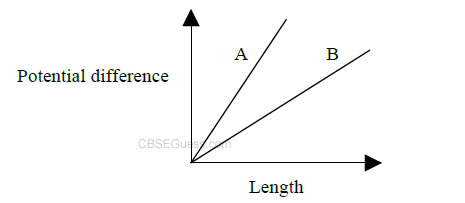# Current Electricity

View Solutions

1. Magnesium is used for making standard resistors, why?

2. The sequence of bands marked on a carbon resistor are: Red, Red, Red, Silver. Write
the value of resistance with tolerance.

3. A wire of resistively ρ is stretched to three its initial length, what will be its new resistively.

4. If p.d.v applied across a conductor is increased to 2v, how will the draft velocity of the electrons change?

5. A 10Ω thick wire is stretched so that its length becomes three times. Assuming that there is no
change in its density on stretching. Calculate the resistance of new wire.

6. You are given 8 Ω resistor. What length of wire of resistance 120 Ωm-1 should be joined in parallel with it to get a value of 6 Ω ?

7. Three resistance 3Ω,6Ω and 9Ω are connected to a battery. In which of them will the power dissipation be maximum if

a) They are all connected in parallel
b) They are all connected in series
Give reason.

8. A silver wire has a resistance of 2.1Ω at 27.5˚c and a resistance of 2.7Ω at 100˚c. determine the temperature coeff. of resistivity of silver.

9. Give any two applications super conductors.

10. Two wire of equal length one copper and manganin have same resistance , which wire is thicker?

11. Why manganin is used for making standard resistor?

12. A copper wire of resistivity r is stretched to reduce its diameter to half of its previous value .What will be the new resistances?

13. The variation of potential difference with length incase of two potentiometres A and B is given below.Which of the two is more sensitive.14. If the length of the wire conductor is doubled by stretching it , keeping potential difference constant by what factor the drift speed of the electron changed.

15. If the temperature of the conductor increases, how does the relaxation time of electron changes.

16. A heater joined in series with the 60W bulb .With change of bulb with 100 W in the circuit, the rate heat produce by the heater will more or less or remain same.

17. What will be the change in the resistance of the circular wire , when its radius is halved and length is reduced by ¼ th of original length.

18. Two 120V light bulbs , one of 25W and another of 200W are connected in series . One bulb burnt out almost instantaneously ?.Which one was burnt and why?.

19. A given copper wire is stretched to reduce its diameter is half of its original value.What will the new resistance?.

20. A student has two wire of iron and copper of equal length and diameter.He first joins two wires in series and pass electric current through the combination which increases gradually.After that he joins two wires in parallel and repeats the process of passing current.Which wire will glow first in each case?

21. A cylindrical metallic wire is stretched to increase its length by 5% . Calculate the percentage change in resistances.

22. A wire of resistance 4R is bend in the form of circle .What is the effective resistance between the ends of diameter?.

23. Two wires A and B have same lengths and material , have their cross sectional areas 1:4 , what would be the ratio of heat produced in these wires when the voltage across each is constant.

24. Two bulbs whose resistance are in the ratio of 1:2 are connected in parallel to a source of constant voltage. What will be the ratio of power dissipation in these?

25. Total resistance of the circuit is R/3 in which three identical resistors are connected in parallel. Find the value of each resistance?.

CBSE Physics (Chapter Wise With Hint / Solution) Class XII (By Mr. Sreekumaran Nair)